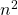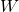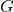# Random walks on quasirandom graphs

Take a long (proportional to) random walkin a quasirandom graph. Must the subgraph of edges traversed bybe quasirandom? We’d like to say yes, for the following reason:visits every vertex about the same number of times, so we pick up the same number of random edges at every vertex. In the case where the minimum degree ofis large, this argument is essentially correct. Ifhas some vertices of very low degree then it breaks down because the random walk can get stuck in clusters of low degree vertices. However, a more sophisticated argument can recover a result that is almost as strong.

The proofs both fall into two parts: first show that the random walk does not differ too much from a process that has much more independence, then exploit that independence by applying standard concentration results to show that things work with high probability. It turns out that our results can be tweaked to apply to the more general case of random homomorphisms of trees (rather than paths) provided the maximum degree of the tree isn’t too large, so we indicate the necessary changes at the end of the paper.